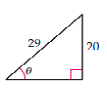Chapter 7.6, Problem 6ES### Mathematical Excursions (MindTap C...

4th Edition
Richard N. Aufmann + 3 others
ISBN: 9781305965584

#### Solutions

Chapter
Section### Mathematical Excursions (MindTap C...

4th Edition
Richard N. Aufmann + 3 others
ISBN: 9781305965584
Textbook Problem

# Find the values of sin θ , cos θ , and tan θ for the given right triangle. Give the exact values.To determine

The values of sin(θ) , cos(θ) and tan(θ) for the given right triangle.

Explanation

Given:

Calculation:

sin(θ)=opposite sidehypotenusesin(θ)=2029cos(θ)=adjacent sidehypotenusecos(θ)= 29 2 20 2 29= 84140029cos(θ

### Still sussing out bartleby?

Check out a sample textbook solution.

See a sample solution

#### The Solution to Your Study Problems

Bartleby provides explanations to thousands of textbook problems written by our experts, many with advanced degrees!

Get Started

## Additional Math Solutions

#### Find more solutions based on key concepts